# On Blasius Plate Solution of Particle Dispersion and Deposition in Human Respiratory Track

On Blasius Plate Solution of Particle Dispersion and Deposition in Human Respiratory Track

Samson Kolawole Fasogbon Funmilayo Helen Oyelami* Emmanuel Oreoluwa Adetimirin Ebenezer Olubunmi Ige

Department of Mathematical and Physical Sciences, Afe Babalola University, Ado Ekiti 360001, Nigeria

Department of Biomedical Engineering, Afe Babalola University, Ado-Ekiti 360001, Nigeria

Corresponding Author Email:
Page:
428-432
|
DOI:
https://doi.org/10.18280/mmep.060314
6 August 2018
|
Accepted:
20 January 2019
|
Published:
15 September 2019
| Citation

OPEN ACCESS

Abstract:

This study investigates combust fuel aerosol contained in the atmosphere and their inhalation and deposition in the human respiratory tract, resulting to Environmental pollution, particularly in developing nations where emission control in vehicular devices are not well enforced. Effects of fuel density on the rate of aerosol deposition on the walls of a model respiratory tracts using two-dimensional consideration of the equations of momentum, energy and convection-advection and also characterized the dispersion and deposition of aerosols from selected combust fossil fuels in the respiratory tract by inertial impaction using relevant dimensionless numbers was considered. Using a similarity variable, the resulting partial differential equations were transformed into self-similar ordinary differential equations and the boundary value problem to an initial-value problem using the concept of shooting and fourth-order Runge-Kutta methods. The results showed that higher density fuels deposited more aerosols on the walls of the respiratory tract with significant reduction in respiratory tracts diameters as the aerosol deposition/concentration continues on the tracts. The study numerically presented deposition behaviour of a variety of fossil-fuel and bio-fuel combusts, deposition pattern of high density fuels deposit was greater in comparison to bio-fuels derived aerosols in the computational domain of the model tract. Thus, bio-fuels are presented as alternate.

Keywords:

combust fuel, Environmental pollution, bio-fuel combusts, combust fossil aerosol, Blasius solution

1. Introduction

The use of alternative sources to generate electricity has become dominant in Nigeria, where the power supply is erratic. The electricity generating sets most commonly used burn gasoline and diesel to produce exhaust gases, and consequently, aerosols that are rich in sulphuric acid, nitrogen oxides and oxides of carbon which all contribute significantly to air pollution . This acid has proven to be a source of environmental pollution. Environmental pollution is one causal factor of Chronic Obstructive Pulmonary Diseases (COPD).

The inhalation of these aerosol particles has become a significant health risk in the environment [2-3] predicted the future emissions of Africa as a compared to the emissions of other continents and suggested that the emissions would increase in consequent years. Africa, as at 2005, accounted for about 3.7 % of global emissions. The emissions were based on combust fossil fuels. The increase of emissions in Africa was 3.2 %, slightly lower than the global average. As a result of population growth, more emissions were predicted in the coming years. This suggests that more fossil fuels will be burned, leading to more emissions and the need to appropriately combat the effect of the emissions on human health. Over the years, the necessity for off-grid private electricity generation in clustered settlement of major cities in Nigeria has resulted into appalling scenarios that have resulted to the death of hundreds of lives.  The suffocating effect caused by sustained low oxygen inhalation and carbon (aerosol) deposition in lung air-ways of the human respiratory systems.

The combustion of fossil fuels pollutes the environment and yields aerosols that travel in the air with the tendency to be inhaled by human beings . Aerosol particles is a global risk factor for healthy individuals while COPD patients are highly susceptible to inhalation of these particulate emission in the atmosphere [3-4] from a study of emission pattern predicted a greater level of pollution in from the combustion of fossil fuels in Africa. Nnaji et al.  and Mahajan  also corroborated the projected increase in pollution from fossil fuel combustion. Hofmann et al.  compared results for deposition for various fuels. The results of the study by these authors indicated that petrol and diesel had significant deposition percentages. The deposition of particles is initiated by three major mechanisms viz. gravitational sedimentation, diffusion and inertial impaction. Various models have been presented to study different deposition mechanisms [7-8].  Size and density of the particles were two major factors affecting deposition . Early studies conducted on the respiratory tract were experimental  and the first three bifurcations were used . The flow in the respiratory airways is generally assumed to be laminar, as turbulent effects are minimal  and particles deposited during respiration are small with the deposition mechanisms depending on the size of the particles. Katiyar  studied the flow pattern for respiration, using Reynolds number between 200 and 1600. Ou et al.  also simulated deposition with Reynolds number ranging from 100 to 2000 and using particle sizes from 1-10 mm. Oakes et al.  on the other hand, studied airflow and particle deposition with consistent particle size. Oresta et al.  investigated the flow and particle dispersion with direct numerical simulation and the Lagrangian particle tracking in a closed slender geometry. The authors varied Stokes number from 0.13-1.3 and Prandtl number taken as 0.7. They found the convection-advection equation to be a valid tool to study deposition.

The flow of air and aerosol is considered as a two-phase flow. The aerosol particles are fine particles travelling in air as a result of burning fossil fuels. Jolgam et al.  researched the equations of state for simulations of multiphase flow. A Eulerian numerical method was considered for simulating multiphase flow governed by the equation of state. This method makes use of the volume fraction in the conservation equations. It employs finding an average density and average velocity for the particles that will be used in the normal conservation equations. The superficial velocity is used for computation. The deposition of fossil fuel generated aerosols was compared, and the deposition over respiratory tracts of different diameters was studied.

Particle deposition consequent of inhalation of combust fossil fuels has been reported in some investigation. Hofmann et al.  compared the experimental results with the theoretical results for the deposition of combustion aerosols in the respiratory tract. The breathing conditions for 14 non-smokers was computed and the specific size distribution for each inhalation experiment. The theoretical predictions for depositions were 34.6 % for petrol and 24.0 % for diesel while the experimental results were 41.4 % for petrol and 29.6 % for diesel. The large difference in the values between the theoretical and experimental values was attributed to varying and growing sizes of aerosols and mechanisms of deposition. Shape, as compared to many other factors like condensation and evaporation was the major factor considered to influence deposition.

Nnaji et al.  studied the relationship between electricity supply, fossil fuel consumption, aerosol emissions and growth in Nigeria between 1971 and 2009. The bound-test approach was used, alongside co-integration. The findings indicated that aerosol emissions increased as economic growth increased also. Also, it was found that the electricity supply is insufficient and has not significantly impacted the economic growth in Nigeria. This suggests that many individuals and organizations will keep burning fossil fuels to carry out their businesses and to live comfortably at home. Mahajan  also studied the pollution caused by internal combustion engines. He emphasized how the pollution will increase due to the higher number of people buying vehicles and also as a result of industrial processes that make use of internal combustion engines.

A combination of inertial impaction and gravitational sedimentation has been taking into consideration but by very few authors. Nicolaou and Zaki  studied the deposition of particles upon rectangular surfaces. They prescribed the term for deposition due to inertial impaction. The prescription was based on Newton’s first law. A model of the simultaneous action of inertial impaction and gravitational sedimentation was presented by Balásházy et al. . Three flow patterns-uniform, radial, rotational-were studied and the deposition efficiencies for these various flows were computed. The contribution of each mechanism of deposition was also observed as a factor of the total deposition in the airway bifurcation. The study indicated that a two dimensional model is sufficiently good to study deposition. The role of dispersion in aerosol deposition in the human airways was also studied by Lee . Dispersion and deposition of aerosols are processes that occur concurrently but dispersion has not been taken into significant consideration. Lee  coupled the understanding of dispersion to a dosimetry model and it was shown that dispersion affects the deposition of the aerosols. Dispersion was discovered to increase deposition.

Simulation of two bifurcations was carried out by Zhang et al.  due to computation requirements. Lin et al.  scanned images of a 19-year-old healthy boy and built a geometric model of the upper respiratory tract. RNG k-e turbulence model was employed to describe the flow and the particle deposition for three breathing conditions-15L/min, 30L/min and 60L/min. Particle size in relation to particle deposition was studied and various sizes of particles from 0.1mm to 8mm were used. The velocities in the respiratory tract were observed to have been increasing from the walls of the tract. The nasal cavity was established to be the region with the highest deposition, followed by the pharynx and larynx. It indicates that the mass transfer was prominent from the entry and gradually decreased across the respiratory tract.

Reynolds number and Stokes number are two of the factors that affect particle deposition. Katiyar  simulated flow with Reynolds number between 200 and 1600. Ou et al.  employed finite-volume based simulations to investigate airflow pattern and the effect of various airflow patterns on particle deposition in human airways. Two particle deposition mechanisms-gravitational sedimentation and impaction-were considered while particle sizes were varied from 1-10mm. The range of Reynolds number used was 100-2000, increasing in steps of 100. Deposition fractions were found to be different for different particles at various Reynolds number. Zhang investigated the effect6 of Stokes number on particle deposition. At lower values of Stokes number, Zhang discovered that high flow rates had lower deposition fractions.

The convection-diffusion is commonly used to model transport of particles in fluids. The equation shows close correlation with the direct numerical simulation data. Turbulent flow and particle dispersion were investigated with direct numerical simulation and the Lagrangian particle tracking in a closed slender geometry by Oresta et al. . The study revealed that advection diffusion equation is a valid tool to study the deposition of aerosols.

The focus of the present investigation is to numerically study the dependence of nature of combust fossil on aerosol deposition in model respiratory air-track. Likewise, the influence of varying combust fossil fuel aerosol on aerosol dispersion in upper respiratory tracks was investigated. Well known Navier-Stokes equations and concentration-diffusion equations were employed and solved to obtain Blassius solution for momentum, concentration and thermal fields. These procedures are presented in the subsequent section of this report.

2. Method/Experiment/Body

The respiratory tract was considered as a flat plate and the flow was considered to be laminar, steady and Newtonian. The deposition mechanism considered was impaction. The deposition of fossil fuel generated aerosols in the respiratory tract occurs at the walls and was considered as a boundary layer problem. It should be noted that the respiratory tract in human is of a very complex geometry, this simplification is needed to for numerical computation. Five fuels were considered in the study viz. coke, diesel, gasoline, kerosene and methane. Methane, gasoline and diesel have densities lower than 1 kg/m3 while coke and kerosene have densities greater than 400 kg/m3. The boundary layer assumption was employed to simplify the problem and obtain an appropriate solution of the Navier-Stokes equation (Eq. 1-3), the energy equation (Eq. 4) and the convection-advection equation (Eq. 5).

$\frac{\partial u}{\partial x}+\frac{\partial v}{\partial y}=0$   (1)

$\rho\left(u \frac{\partial u}{\partial x}+v \frac{\partial u}{\partial y}\right)=-\frac{\partial P}{\partial x}+\rho g_{x}+\mu\left(\frac{\partial^{2} u}{\partial x^{2}}+\frac{\partial^{2} u}{\partial y^{2}}\right)$     (2)

$\rho\left(u \frac{\partial v}{\partial x}+v \frac{\partial u}{\partial y}\right)=-\frac{\partial P}{\partial y}+\rho g_{y}+\mu\left(\frac{\partial^{2} v}{\partial x^{2}}+\frac{\partial^{2} v}{\partial y^{2}}\right)$     (3)

$u \frac{\partial T}{\partial x}+v \frac{\partial T}{\partial y}=\alpha\left(\frac{\partial^{2} T}{\partial x^{2}}+\frac{\partial^{2} T}{\partial y^{2}}\right)$        (4)

$u \frac{\partial c}{\partial x}+v \frac{\partial c}{\partial y}=D\left(\frac{\partial^{2} c}{\partial x^{2}}+\frac{\partial^{2} c}{\partial y^{2}}\right)+R$   (5)

The order of magnitude analysis was performed to determine the terms that have significant effects, keeping those and neglecting the effects of the terms with very small magnitudes and yielded equations (6-8).

$u \frac{\partial u}{\partial x}+v \frac{\partial v}{\partial y}=v \frac{\partial^{2} u}{\partial y^{2}}$         (6)

$u \frac{\partial T}{\partial x}+v \frac{\partial T}{\partial y}=\alpha \frac{\partial^{2} T}{\partial y^{2}}$            (7)

$u \frac{\partial c}{\partial x}+v \frac{\partial c}{\partial y}=D \frac{\partial^{2} c}{\partial y^{2}} R$        (8)

Introducing a stream function, $\Psi$, Let $u=\frac{\partial \Psi}{\partial y}$ and $v=\frac{-\partial \Psi}{\partial x}$ And a similarity variable,

$\eta=\frac{y}{g(x)}, \quad f=\frac{\Psi(x, y)}{h(x)}$      (9)

The dimensionless temperature and concentration were taken as

$\theta=\frac{T-T 1}{T 2-T 1} ; \quad \phi=\frac{c-c 1}{c 2-c 1}$     (10)

Appropriate substitution yielded

$2 f^{\prime \prime \prime}+f f^{\prime \prime}=0 ; f(0)=0, f^{\prime}(0)=0, f^{\prime}(\infty)=1$  (11)

$2 \theta^{\prime \prime}+\operatorname{Pr} f \theta^{\prime}=0$

$\theta(0)=0, \theta(\infty)=1$  (12)

$2 \phi^{\prime \prime}+\mathrm{Sc} f \phi^{\prime}+\mathrm{ScR}=0$

$\phi(0)=1, \phi(\infty)=0$  (13)

But the deposition due to inertial impaction was defined as

$R=\frac{C d R e(U p-U f) f^{\prime}}{48 k}$     (14)

The equation (13) then became

$2 \phi^{\prime \prime}+\operatorname{Sc} f \phi^{\prime}+\frac{2 S c \cdot c d R e(U p-U f) f^{\prime}}{48 k}=0$ (15)

The ordinary differential equations were higher-order equations; hence they were broken down to the respective number of first-order equations, to enable coding in MATLAB 2012b. The solution was obtained and the concentration profiles and gradients were compared for various fuels.

3. Results/ Discussion

3.1 Investigation of aerosol dispersion for variety of combust fossil fuel

The concentration and concentration profiles are plotted for varying values of Schmidt numbers. Figure 1 shows a graph of the concentration profile against h at different values of Schmidt numbers. Schmidt number is a ratio of the momentum diffusivity to mass transfer. Hence, as mass transfer increases, the concentration profile reduces. Therefore, if the momentum diffusivity is much smaller than the mass transfer, the concentration profile increases for constant values of h. Figure 2 shows the concentration gradient for various Schmidt numbers. It is observed that the magnitude of the concentration gradient initially decreases with increasing Sc till h reaches about 8, it then starts to increase as Sc increases.

## 1.png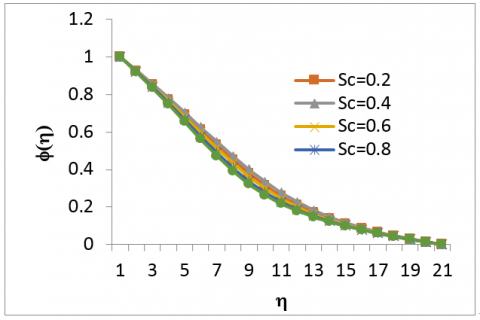Figure 1. Concentration profile for various Sc

## 2.png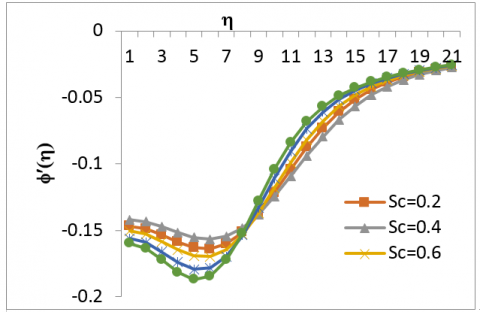Figure 2. Concentration gradient profile for various values of Sc

## 3.png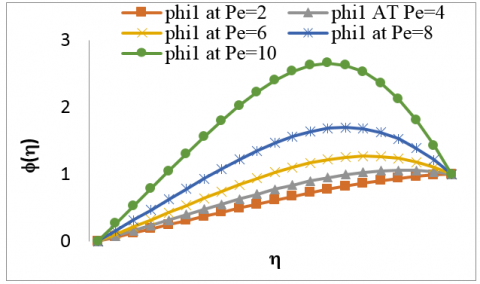Figure 3. Concentration Profile over various values of Pe

Figures 3 and 4 show the concentration and concentration gradient profiles for different values of Peclet numbers. As Pe increases, the concentration profile increases till h reaches 11 then the concentration profile begins to reduce. The concentration gradient decreases for higher values of Pe till h reaches 11 and it starts to increase. Pe number can be explained as the rate of dispersion to diffusion. Hence, when dispersion becomes the prominent mechanism, the concentration profile is higher than when it is diffusion. Also, mass transfer is lower for dispersion till it gets to a point when it increases and becomes higher as compared to diffusion.

## 4.png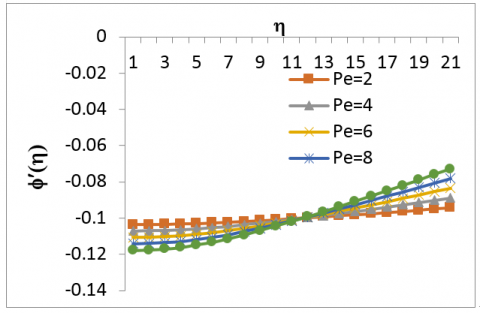Figure 4. Concentration gradient profiles for different values of Pe

3.2 Investigation of aerosol deposition for a variety of combust fossil fuel

The concentration and concentration gradients of aerosols generated by different fuels are plotted as the boundary layer thickness increases.

The effect of aerosol particles generated by gasoline was studied over different airway diameters. In Figure 5, the concentration profile of the aerosol particles was higher for smaller airway diameters, at any distance from the wall of the respiratory tract. The concentration of the aerosols decreased rapidly for airways with large diameters.

## 5.pngFigure 5. Concentration Profile for Various sizes of Respi rssatory tract

## 6.png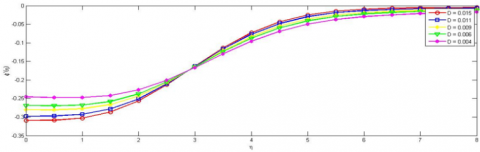Figure 6. Concentration gradient Profile for Various sizes of respiratory tract

The concentration gradient profile in Figure 6 shows that tracts with larger diameters experienced more deposition close to the wall. At η=6.5, the airways with smaller diameters started to experience more deposition. From the concentration profile in Figure 3, at η=6.5, the concentration of the airway with least diameter was 40 % of the initial value while that of the largest diameter was about 20 % of the initial value.

4. Conclusions

Ordinary differential equations were derived from the partial differential equations that described the motion of the fluid. The ordinary differential equations were solved with the fourth order Runge-Kutta method. Satisfactory results were obtained and this study shows that higher density fuels dispersed more aerosol particles at the walls of the respiratory tract and pose a greater threat to the health of human beings. Airways with larger diameters experienced more deposition. At lower concentrations, fuels with lower densities and the smaller airways experienced more deposition. For the investigation of deposition study, mass diffusivity and diffusion were found not to contribute significantly to deposition. Aerosol deposition was found to increase as momentum diffusivity became more prominent. Aerosol deposition also increased as the effect of dispersion became more significant. This study shows that momentum diffusivity and dispersion aid aerosol deposition at the walls of the respiratory tract.

References

 Abm, S.K., Nigel, N.C., Gregory, J.T., Wayne, W.S., Gautam, M., Lyons, D.W. (2006). Idle Emissions from heavy-duty diesel vehicles: Review and recent data. Journal of Air &Waste Management Association, 56: 1404-1419. https://doi/abs/10.3155/1047-3289.59.3.354

 Theunissen, R., Buchmann, N., Corieri, P., Riethmuller, M.L., Darquenne, C. (2006). Experimental investigation of aerosol deposition in alveolar lung airways. 13th International Symposium on Applications of Laser Techniques to Fluid Mechanics Lisbon, Portugal, Paper (1098): 1-2.

 Canadell, J.G., Raupach, M.R., Houghton, R.A. (2009). Anthropogenic CO2 emissions in Africa. Biogeosciences, 6: 463-468. https://doi.org/10.5194/bg-6-463-2009

 Nnaji, C., Chukwu, J., Moses, N. (2013). Electricity supply, fossil fuel consumption, CO2 emissions and economic growth: Implications and policy options for sustainable development in Nigeria. International Journal of Energy Economics and Policy, 3(3): 262-271.

 Mahajan, S.R. (2013). Air pollution from I.C. engines & it’s control. International Journal of Inventive Engineering and Sciences (IJIES), 1(11): 1-4.

 Hofmann, W., Morawska, L., Winkler-Heil, R., Moustafa, M. (2009). Deposition of combustion aerosols in the human respiratory tract: comparison of theoretical predictions with experimental data. Inhalation Toxicology, 21(14): 1154-1164. https://doi.org/10.3109/0895837090280669

 Stahlhofen, W., Rudolph, G., James, A.C. (1989). Intercomparison of experimental regional aerosol deposition data. Journal of Aerosol Medicine, 2(3): 285-308. https://doi/abs/10.1089/jam.1989.2.285

 Asgharian, B., Menache, M.G., Miller, F.J. (2004). Modeling age-related particle deposition in humans. Journal of Aerosol Medicine, 17(3): 213-224. https://doi.org/10.1089/jam.2004.17.213

 Pilcer, G., Amighi, K. (2010). Formulation strategy and use of excipients in pulmonary drug delivery. International Journal of Pharmacy, 392: 1-19. https://doi.org/10.1016/j.ijpharm.2010.03.017

 Jin, H.H., Fan, J.R., Zeng, M.J. (2007). Large eddy simulation of inhaled particle deposition withinthehuman upper respiratory tract. Journal of Aerosol Science, 38(3): 257-268. https://doi.org/10.1016/j.jaerosci.2006.09.008

 Ochs, M., Jens, R., Nyengared, J.A. (2004). The number of alveloli in the human lung. American Journal of Respiratory. Critical Care Medicine, 169: 120-124. https://doi.org/10.1164/rccm.200308-11070c

 Xi, J., Longest, P.W., Martonen, T.B. (2008). Effects of the laryngeal jet on nano- and microparticle transport and deposition in an approximate model of the upper tracheobronchial airways. Journal of Applied Physiology, 104: 1761-1777. https://doi.org/10.1152/japplpysiol.01233.2007

 Katiyar, V.K. (2012). Numerical simulation of flow structure and deposition of particles in asthematic airway bifurcation. International Journal of Enhanced Research in Science Technology & Engineering, 1(3): 1-5.

 Ou, C., Deng, Q., Liu, W. (2012) Numerical simulation of particle deposition in obstructive human airways. Journal of Central South University of Technology, 19(3): 609-614. https://doi.org/10.1007/s11771-012-1046-x

 Oakes, J., Marsden, A.L., Grandmont, C., Shadden, S.C., Darquenne, C. (2014). Airflow and particle deposition simulations in health and emphysema: From in vivo to in silico animal experiments. Annals of Biomedical Engineering, 42(4): 899-914. https://doi.org/10.1007/s10439-013-0954-8

 Oresta, P.A., Roberto, V., Alfredo, S. (2005). Advection diffusion model for particles deposition in Rayleigh- Bénard turbulent flows. International Conference Nuclear Energy for New Europe, Bled, Slovenia, 173: 1-15.

 Jolgam, S., Ballil, A., Nowakowski, A., Nicolleau, F. (2012). On equations of state for simulations of multiphase flows. Proceedings of the World Congress on Engineering, 3: 1.

 Nnaji, C., Chukwu, J., Moses, N. (2013). Electricity supply, fossil fuel consumption, CO2 emissions and economic growth: Implications and policy options for sustainable development in Nigeria. International Journal of Energy Economics and Policy, 3(3): 262-271.

 Nicolaou, L., Zaki, T.A. (2013). Direct numerical simulations of ﬂow in realistic mouth-throat geometries. Journal of Aerosol Science, 57: 71-87. https://doi.org/10.1016/j.jaerosci.2012.10.003

 Balásházy, I., Martonen, T.B., Hofmann, W. (1990). Inertial impaction and gravitational deposition of aerosols in curved tubes and airway bifurcations. Aerosol Science and Technology, 13(3): 308-321. https://doi.org/10.1080/02786829008959447

 Lee, D.Y., Lee, J.W. (2002). Dispersion of aerosol bolus during one respiration cycle in a model of lung airways. Journal of Aerosol Science; 333: 1219-1234. https://doi.org/10.1016/s0021-8502(02)00053-8

 Zhang, L.Z., Cheng, H.J., Zhang, C.B., Xu, Z.J., Ye, J. (2006). Deposition fraction of aerosol particles in a human oral airway model on stable condition. Aerosol and Air Quality Research, 6(3): 259-267. https://doi.org/10.4209/aaqr.2006.09.0003

 Lin, J., Hu, G.L., Fan, J.R., Pan, D. (2009). Study on airflow and inhaled particle deposition within realistic human upper respiratory tract. The 6th International Symposium on Measurement Techniques for Multiphase Flows, Journal of Physics: Conference Series, p. 147.